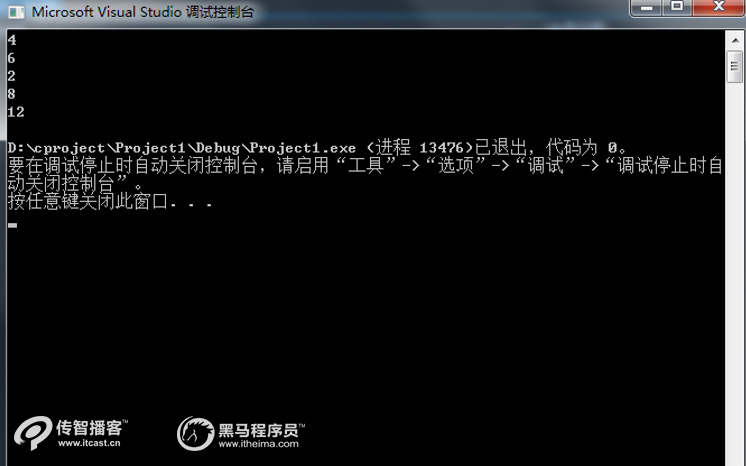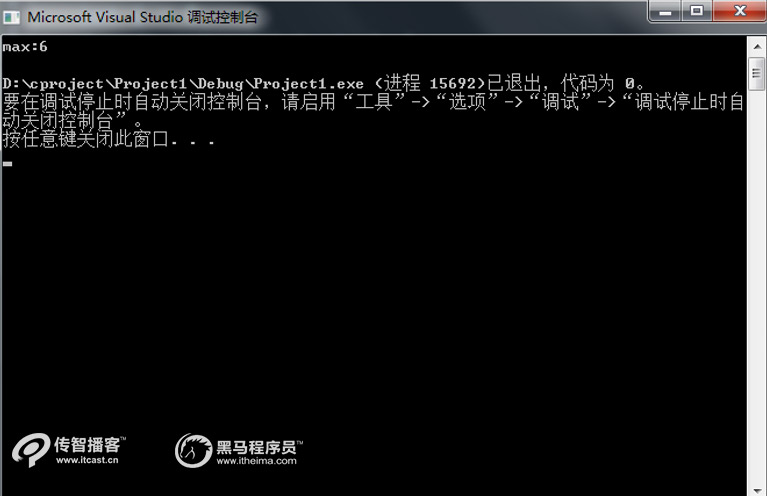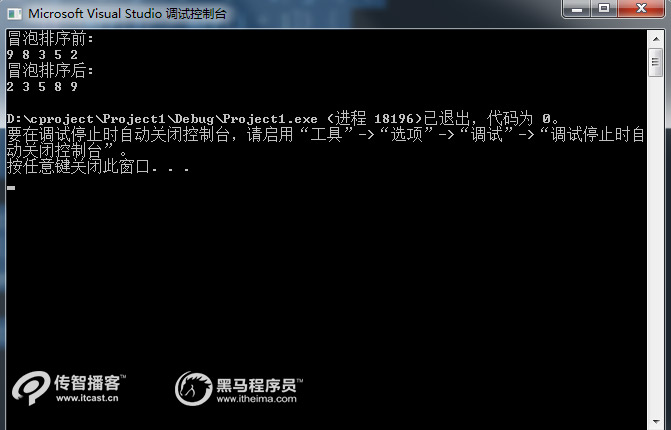# C++中一維數組是什么？怎么使用？【C++培訓】

int array[5l;

（1）直接對數組中的所有元素賦值，示例代碼如下：

int i={1,2,3,4,5};

（2）只對數組中的一部分元素賦值，示例代碼如下

int i={1,2,3};

（3）對數組全部元素賦值，但不指定長度，示例代碼如下：

int i[]={1,2,3,4};

（1）數組的下標是用方括號括起來的，而不是圓括號；

（2）數組名的命名同變量名的命名規則相同；

（3）數組定義中，常量表達式的值可以是符號常量，如下面的定義就是合法的。

int a[N];      //假設預編譯命令#define N 4，下標是符號常量

#include

void main(int argc, char *argv[]){

int x = {2,3,1,4,6};

int i;

for (i = 0; i < 5; i++) {

printf("%d\n", 2 * x[i]);

}

}1、一維數組的遍歷

#include

void main(int argc, char *argv[]){

int x = {2,3,1,4,6};

int i = 0;

for (i = 0; i < 5; i++) {

printf("x[%d]:%d\n", i, x[i]);

}

}2、一維數組的最值

#include

int main(int argc, char *argv[]){

int x = {2,3,1,4,6};

int nMax = x;

int i = 0;

for (i = 0; i < 5; i++) {

if (x[i] > nMax) {

nMax = x[i];

}

}

printf("max:%d\n", nMax);

return 0;

}3、一維數組的排序

#include

void main(int argc, char ** argv[]){

int arr = { 9,8,3,5,2 };

int temp = 0;

int i, j;

printf("冒泡排序前:\n");

for (i = 0; i < 5; i++) {

printf("%d ", arr[i]);

}

printf("\n");

for (i = 0; i < 5 - 1; i++) {

for (j = 0; j < 5 - 1 - i; j++) {

if (arr[j] > arr[j + 1]) {

temp = arr[j];

arr[j] = arr[j + 1];

arr[j + 1] = temp;

}

}

}

printf("冒泡排序后:\n");

for (i = 0; i < 5; i++) {

printf("%d ", arr[i]);

}

printf("\n");

}C語言如何獲取隨機數?
C/C :指針數組和數組指針淺析
C語言二維數組的定義和引用